# High School Math : How to find the angle of a sector

## Example Questions

### Example Question #46 : Plane Geometry

Solve for.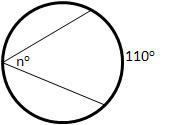(Figure not drawn to scale).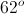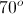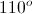Explanation:

We can solve for the angle,, by using the below relationship.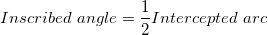In the figure, intercepted arc is given.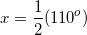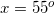### Example Question #47 : Plane Geometry

A pie has a diameter of 12". A piece is cut out, having a surface area of 4.5π. What is the angle of the cut?

12.5°

25°

45°

4.5°

90°

45°

Explanation:

This is simply a matter of percentages. We first have to figure out what percentage of the surface area is represented by 4.5π. To do that, we must calculate the total surface area. If the diameter is 12, the radius is 6. Don't be tricked by this!

A = π * 6 * 6 = 36π

Now, 4.5π is 4.5π/36π percentage or 0.125 (= 12.5%)

To figure out the angle, we must take that percentage of 360°:

0.125 * 360 = 45°

### Example Question #48 : Plane Geometry

Eric is riding a Ferris wheel. The Ferris wheel has 18 compartments, numbered in order clockwise. If compartment 1 is at 0 degrees and Eric enters compartment 13, what angle is he at?

300

280

260

240

180

240

Explanation:

12 compartments further means 240 more degrees. 240 is the answer.

360/12 = 240 degrees

### Example Question #181 : High School Math

Solve for.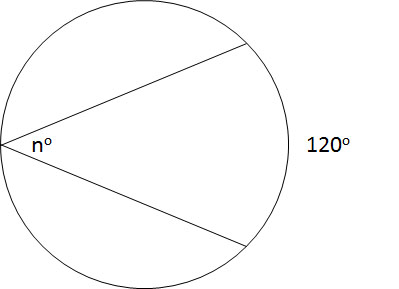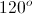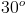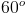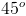Explanation:

The measure of an arc of an inscribed angle is twice the size of the inscribed angle.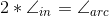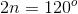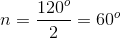### Example Question #1 : How To Find The Angle Of A Sector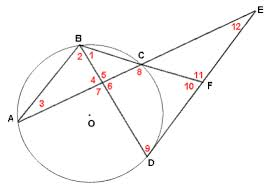In the figure above that includes Circle O, the measure of angle BAC is equal to 35 degrees, the measure of angle FBD is equal to 40 degrees, and the measure of arc AD is twice the measure of arc AB. Which of the following is the measure of angle CEF? The figure is not necessarily drawn to scale, and the red numbers are used to mark the angles, not represent angle measures.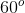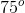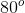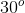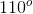Explanation:

The measure of angle CEF is going to be equal to half of the difference between the measures two arcs that it intercepts, namely arcs AD and CD.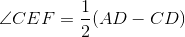Thus, we need to find the measure of arcs AD and CD. Let's look at the information given and determine how it can help us figure out the measures of arcs AD and CD.

Angle BAC is an inscribed angle, which means that its meausre is one-half of the measure of the arc that it incercepts, which is arc BC.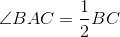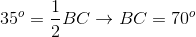Thus, since angle BAC is 35 degrees, the measure of arc BC must be 70 degrees.

We can use a similar strategy to find the measure of arc CD, which is the arc intercepted by the inscribed angle FBD.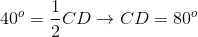Because angle FBD has a measure of 40 degrees, the measure of arc CD must be 80 degrees.

We have the measures of arcs BC and CD. But we still need the measure of arc AD. We can use the last piece of information given, along with our knowledge about the sum of the arcs of a circle, to determine the measure of arc AD.

We are told that the measure of arc AD is twice the measure of arc AB. We also know that the sum of the measures of arcs AD, AB, CD, and BC must be 360 degrees, because there are 360 degrees in a full circle.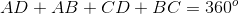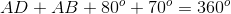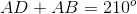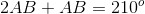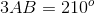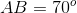This means the measure of arc AB is 70 degrees, and the measure of arc AD is 2(70) = 140 degrees.

Now, we have all the information we need to find the measure of angle CEF, which is equal to half the difference between the measure of arcs AD and CD.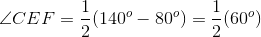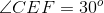### Example Question #21 : Circles

The length of an arc,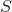, of a circle is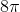and the radius,, of the circle is. What is the measure in degrees of the central angle,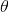, formed by the arc?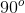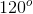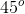Explanation:

The circumference of the circle is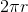.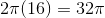The length of the arc S is.

A ratio can be established: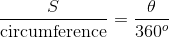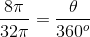Solving foryields 90o

Note: This makes sense. Since the arc S was one-fourth the circumference of the circle, the central angle formed by arc S should be one-fourth the total degrees of a circle.

### Example Question #2 : How To Find The Angle Of A Sector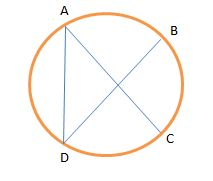In the circle above, the length of arc BC is 100 degrees, and the segment AC is a diameter. What is the measure of angle ADB in degrees?

100

40

90

80

cannot be determined

40

Explanation:

Since we know that segment AC is a diameter, this means that the length of the arc ABC must be 180 degrees. This means that the length of the arc AB must be 80 degrees.

Since angle ADB is an inscribed angle, its measure is equal to half of the measure of the angle of the arc that it intercepts. This means that the measure of the angle is half of 80 degrees, or 40 degrees.

### Example Question #23 : Circles

What is the angle of a sector of area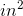on a circle having a radius of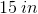?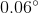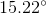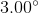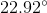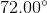Explanation:

To begin, you should compute the complete area of the circle: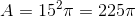Now, to find the angle measure of a sector, you find what portion of the circle the sector is. Here, it is: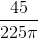Now, multiply this by the total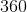degrees in a circle: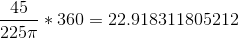Rounded, this is.

### Example Question #11 : Sectors

What is the angle of a sector that has an arc length of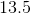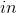on a circle of diameter?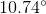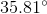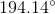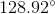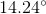Explanation:

The first thing to do for this problem is to compute the total circumference of the circle. Notice that you were given the diameter. The proper equation is therefore: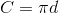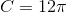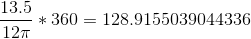Rounded to the nearest hundredth, this is.